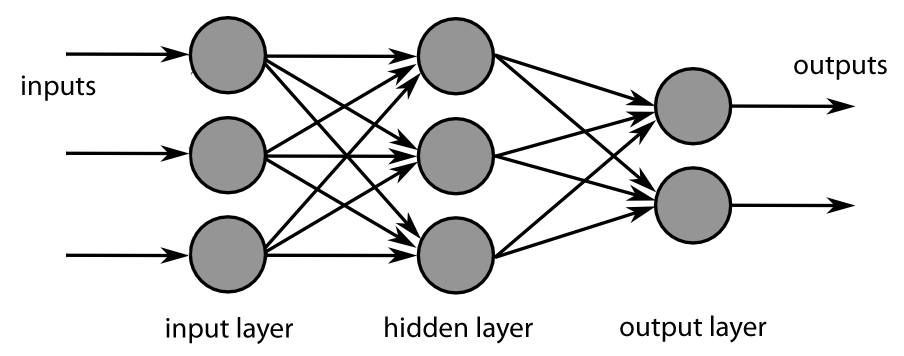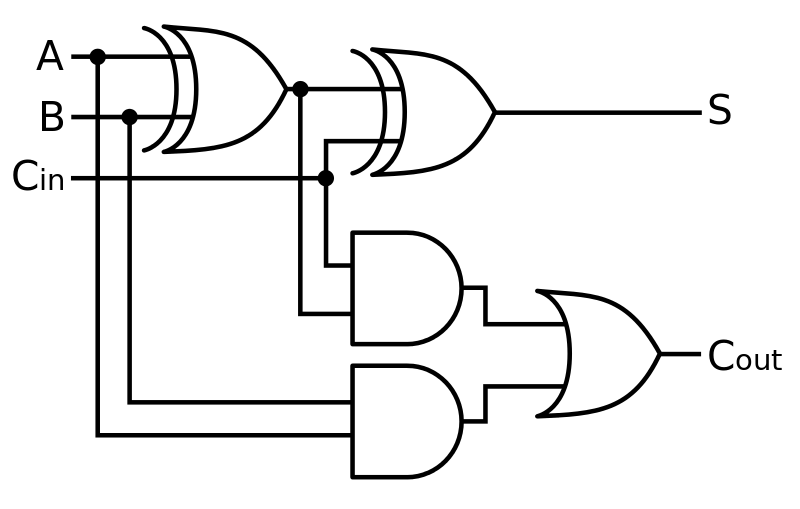## One-Hot Encoding

In computer science and electronics, there are quite a few ways to represent data, often called encoding schemes. Each has its own purpose, advantages, and disadvantages. In this short article we'll take a look at one-hot encoding and see what it is, how it compares to other similar schemes, and its applications.

### What is One-Hot Encoding?

One-hot encoding is a sparse way of representing data in a binary string in which only a single bit can be 1, while all others are 0. This contrasts from other encoding schemes, like binary and gray code, which allow multiple multiple bits can be 1 or 0, thus allowing for a more dense representation of data.

A few examples of a one-hot encoding might look like this:

000010
001000
100000
000100


This concept can better be understood by comparing the representation of the numbers 0-4 in binary, gray code, and one-hot:

Decimal Binary Gray code One-hot
0 000 000 0000000
1 001 001 0000001
2 010 011 0000010
3 011 010 0000100
4 100 110 0001000
5 101 111 0010000
6 110 101 0100000
7 111 100 1000000

Notice how more digits are needed than in the other two representations. Each digit location can only refer to a single number and can't be used to represent any other numbers. Because of this, a one-hot encoding of n digits can only represent n values. Whereas the binary encoding, for example, with n digits can represent 2n values.

### Applications of One-Hot Encoding

So if one-hot encoding has such a strict limit on the amount of data that can be conveyed in a binary string, what practical uses does it actually have? Quite a few important ones, actually. Its importance is explained well by Wikipedia:

One-hot encoding is often used for indicating the state of a state machine. When using binary or Gray code, a decoder is needed to determine the state. A one-hot state machine, however, does not need a decoder as the state machine is in the nth state if and only if the nth bit is high.

#### Machine Learning

Neural networks and deep learning have become the fastest growing discipline in the field of computer science, and for good reason. These machine learning methods have provided a solution to historically difficult, or even impossible, problems in computer science. And, maybe surprisingly, one-hot encoding has an important part in it.

Neural networks, and thus deep learning, are inspired by the neural networks that compose our brains. They consist of simple neurons and connections between the neurons. The output from each neuron usually represents a number, which is used throughout the network for computation. While this number can theoretically have any value, it is usually cast to a 1 or 0 in order to represent categorical data.

For example, let's say we have a neural network that can tell us if an input image is of a cat or a dog. Since the neural network only uses numbers, it can't output the words "cat" or "dog". Instead, it uses one-hot encoding to represent is prediction:In this example, the output of the top neuron in the image above might represent the prediction of "cat" and the bottom neuron's output might represent the prediction of "dog". Whichever output is a 1 would indicate the prediction.

#### Electronics

In electronics, many of the times one-hot encoding is used for very similar scenarios as in machine learning. An analog or digital output line can represent a value using voltage.

For example, logic gate circuits are much like neural networks in that they are composed of a large network of interconnected "nodes", with digital inputs creating some corresponding digital output. While binary representation is common in electronics, one-hot can be a better alternative to represent a an output state without the need for any decoding.